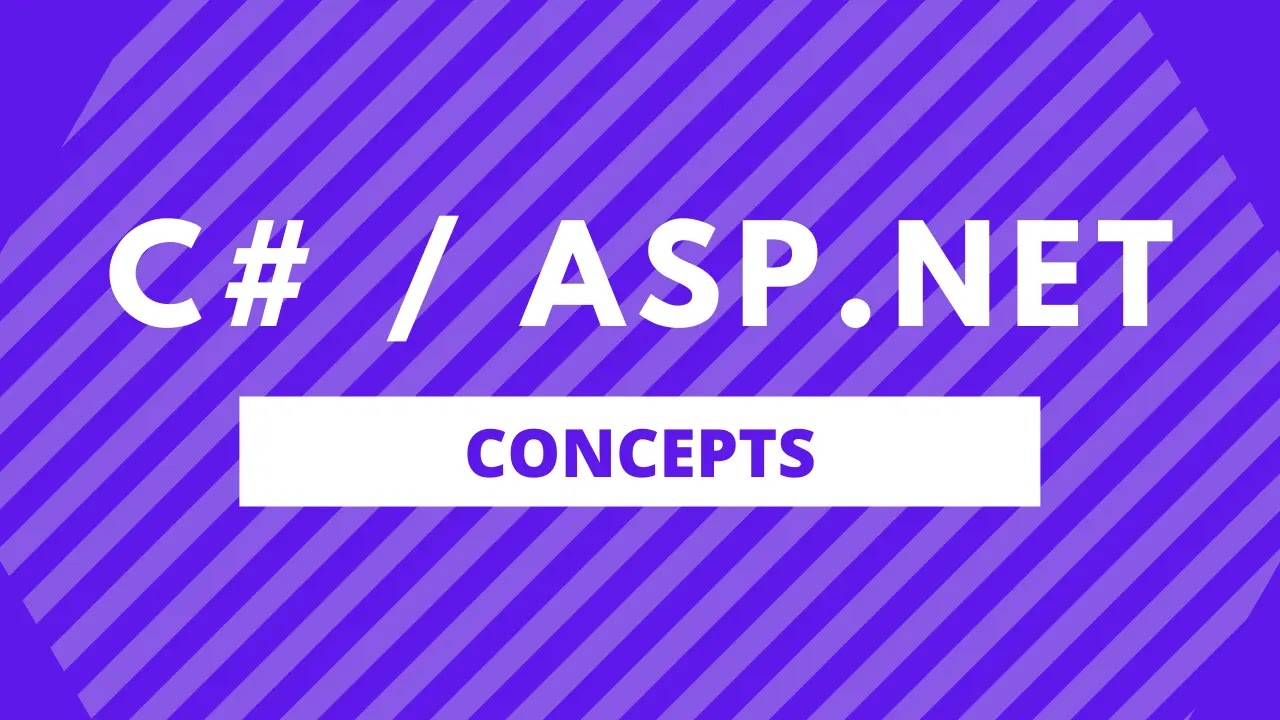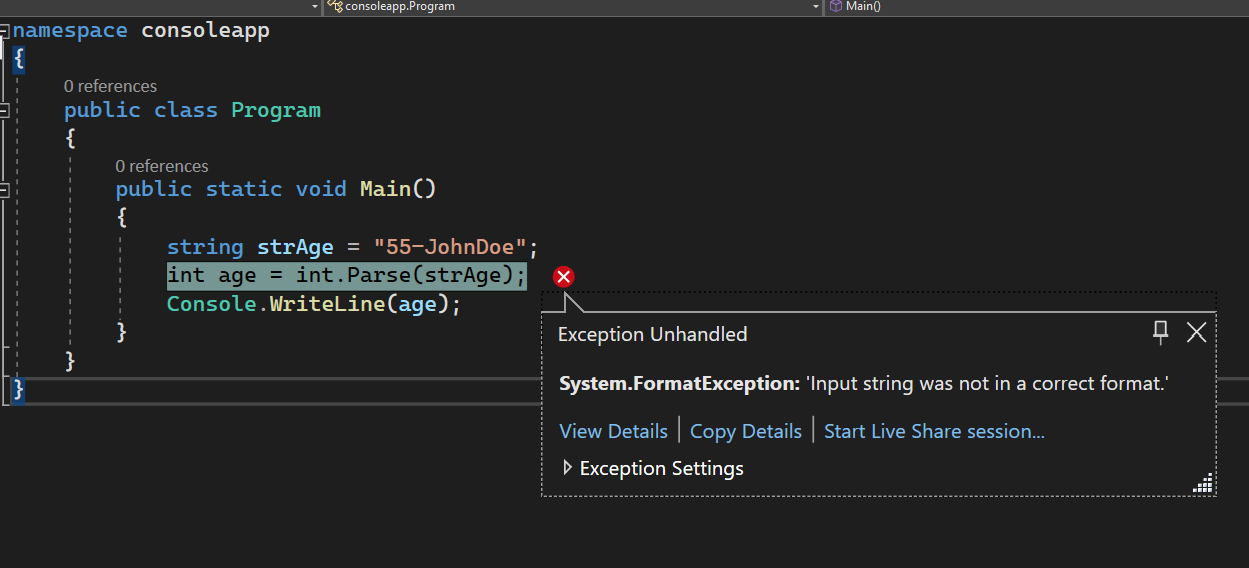# Check If String Is A Number In C#

Sameer Saini July 28, 2022In this blog post, we will learn how to check if a string is a proper number or integer in C#.

Let's say you have a code in which you are getting a age as a string from a database call and you want to check if this string is a number or not before you could use it, this is where this check would be handy.

## Check If String Is A Number In C# Using `int.Parse()` Method

In this method, we will use the `int.Parse()` method and check if the string is a number or not.

The int.Parse() method takes a string as a parameter and then outputs the integer value if the string is a valid number.

If the string is not a valid number or integer, the method throws the exception telling us that this string is not a number.

Let's see this in an example.

Example:

namespace consoleapp { public class Program { public static void Main() { string strAge = "55"; int age = int.Parse(strAge); Console.WriteLine(age); } } }

Result:

55

In the above code example, we can see that we want to check if the value in the string is a valid number or not.

We are using the int.Parse() method to get the int output and writing that to the console.

Let's see another example in which the string was not a valid number.

Example:

namespace consoleapp { public class Program { public static void Main() { string strAge = "55-JohnDoe"; int age = int.Parse(strAge); Console.WriteLine(age); } } }

Result:## Check If String Is A Number In C# Using `int.TryParse()` Method

In this method, we will use the `int.TryParse()` method and check if the string is a number or not.

This method takes in the string as a parameter and gives an out parameter of an integer.

If the string to int conversion was successful, it populates the out parameter with the number value and returns a boolean of true, sugesting that the conversion was sussessful.

If the conversion failed because the string was not a valid number, then the int.TryParse() method returns a boolean of false and the out parameter value as the default integer value, i.e. 0.

Let's see this in an example.

Example:

namespace consoleapp { public class Program { public static void Main() { string strAge = "55"; bool checkResult = int.TryParse(strAge, out int age); if (checkResult == true) { Console.WriteLine(\$"String is a valid number. The value is: {age}"); } else { Console.WriteLine("String is not a valid number."); } } } }

Result:

String is a valid number. The value is 55

If this was string was not a valid number then unlike the Parse method, we would gracefully be able to handle the result and make a decision.

Example:

namespace consoleapp { public class Program { public static void Main() { string strAge = "55-JohnDoe"; bool checkResult = int.TryParse(strAge, out int age); if (checkResult == true) { Console.WriteLine(\$"String is a valid number. The value is: {age}"); } else { Console.WriteLine("String is not a valid number."); } } } }

Result:

String is not a valid number.